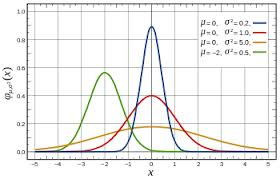## PELIN'S MATHEMATICS PAGE

In mathematics a Gaussian Distribution Function is a function of the form:

In mathematics a Gaussian Distribution Function is a function of the form:

 Distribution Functional Form Mean Standard Deviation Ganussian fg(x)= $\frac{1}{\sqrt{2\pi {\sigma }_{}^{2}}}{e}^{\frac{-{\left(x-a\right)}^{2}}{2{\sigma }_{}^{2}}}$ a ${\sigma }_{\begin{array}{l}\\ \end{array}}$

The Gaussian distribution is one of the most commonly used probability distribution for applications. If the number of events is very large, then the Gaussian distribution function may be used to describe physical events. The Gaussian distribution is a continuous function which approximates the exact binomial distribution of events :

$P\left(y\right)=\frac{1}{\sigma \sqrt{2\pi }}e{\frac{-\left(y-\mu \right)}{2{\sigma }^{2}}}^{2}$

$\mu$:mean of distribution     ${\sigma }^{2}$:variance of the distribution    y is a continuous variable $\left(-\infty \le y\le \infty \right)$

Probability (P) of y being in the range [a, b] is given by an integral

$P\left(yTotal area under the curve is normalized to one.

The probability integral: $P\left(-\infty

The Gaussian distribution is also commonly called the "normal distribution" and is often described as a "bell-shaped curve".The Diagram shows Normalized Gaussian curves with expected value ${\mu }_{}$ and variance ${\sigma }_{}^{2}$ The corresponding parameters are a= $\frac{1}{\left(\sigma \sqrt{\left(2\pi \right)\right)}}$, b= $\mu$, c= $\sigma$. 

The normal distribution with mean 0 and standard deviation 1 N(0;1)is called the standard normal distribution.

A random variable with the standard normal distribution is called a standard normal random variable and is usually denoted by Z.

The cumulative probability distribution of the standard normal distribution $P\left(Z\le z\right)$

has been tabulated and is used to calculate probabilities for any normal random variable.$P\left(Z\le {z}_{0}\right)$ gives the area under the curve to the left of ${z}_{0}$

$P\left({z}_{0}\le Z\le {z}_{1}\right)=P\left(Z\le {z}_{1}\right)-P\left(Z\le {z}_{0}\right)$

The distribution is symmetric $P\left(Z\le {z}_{0}\right)=P\left(Z\ge -{z}_{0}\right)$

Example: Look at the graph below and suppose Z is a standard random variable. Calculate:$\begin{array}{l}P\left(Z\le 1.1\right)\\ P\left(Z>0.8\right)\\ P\left(Z\le -1.52\right)\\ P\left(0.4\le Z\le 1.32\right)\\ P\left(-0.2\le Z\le 0.34\right)\end{array}$

### If you have a question send e-mail:

Name:

E-mail:

Question:

ONLINE QUIZ ABOUT GAUSSIAN DISTRIBUTION

Click here to go to next page: Real life applications of Gaussian Distribution

Click here to go to previous page: Carl Friedrich Gauss